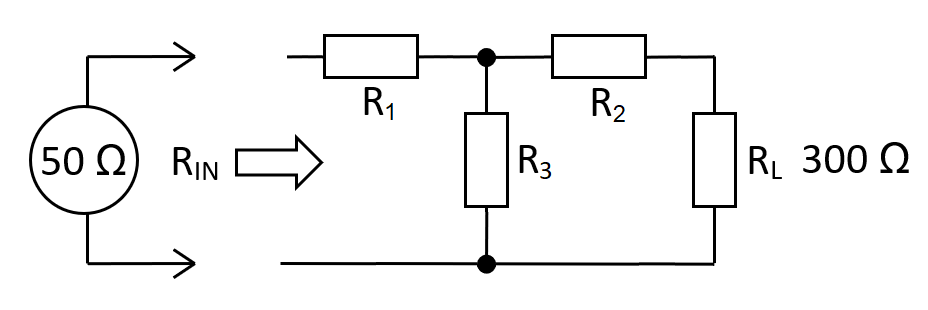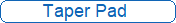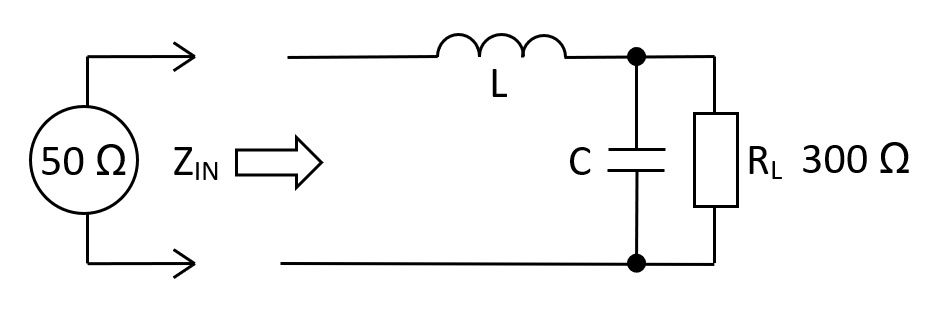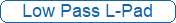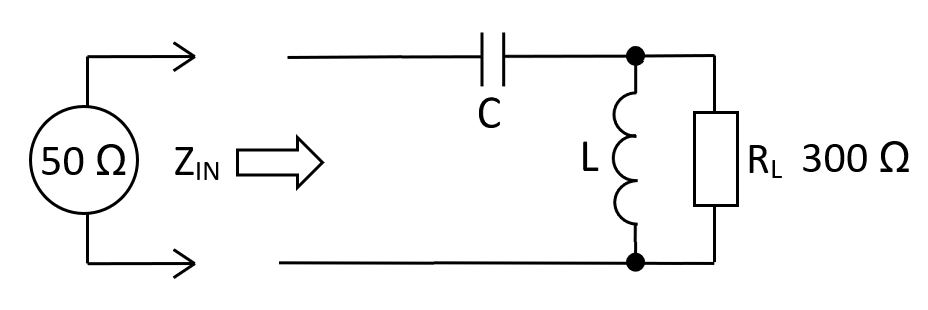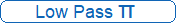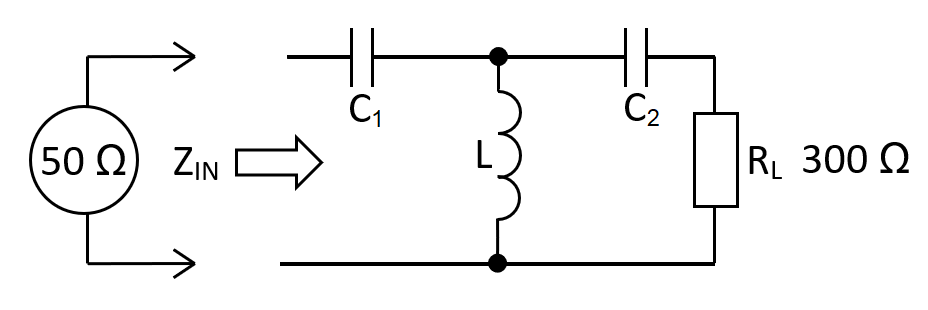When matching impedances, it is not always economical to use a wound transformer. For instance, if the power loss is trivial, two resistors will normally be the right approach. However, if the power loss is significant, then another circuit (the L-pad) can match impedances with minimal power loss.

If moderate spectrum shaping is important, a $$\pi$$ or T network might be more appropriate. If significant spectrum shaping is important then cascading $$\pi$$ or T networks is a good approach.

$$\hspace{18.9cm}$$